Contents (click to collapse) >>

# Clock And Timestamp

## 3 Terminology

item meaning note
hb happened-before
⇒ implies
∧ logical AND
e ∥ f event e and f are concurrent
VC vector clock
LC logical clock
HLC hybrid logical clock
TT true time
PT/pt physical/machine time
NTP network time protocol
QPS timestamp query per second

## 4 Timestamp Oracle Service

Timestamp Oracle Service (TOS), this name was introduced in Google’s percolator, is a centralized service, it is responsible for generating timestamps for the whole distributed system, all timestamps are unique, the timestamps it generates can be logical (counter) or “hybrid” (machine time + counter).

This may be the most simple way to manage timestamp, however, it has several disadvantages, because it’s centralized:

1. may be hard to keep the system with high availability, once the TOS is down, the whole system is down
2. not suitable for cross-DC distributed systems due to high network latency
3. not extendible for high QPS due to only 1 machine can serve## 5 True Time

TrueTime is a global synchronized clock with bounded non-zero error: it returns a time interval that is guaranteed to contain the clock’s actual time for some time during the call’s execution. Thus, if two intervals do not overlap, then we know calls were definitely ordered in real time.

TrueTime是Google Spanner采用时钟算法(Marzullo Algorithm), 基于GPS+原子钟等特殊 硬件, 实现成本较高本质上类Physical Clock时钟算法思路, 以一个误差区间来代替时刻 点. TrueTime 时钟系统精度远高于NTP, 可将时延控制在10ms或更低以内, 并 且在2012年就宣称可以做到1ms以内的误差.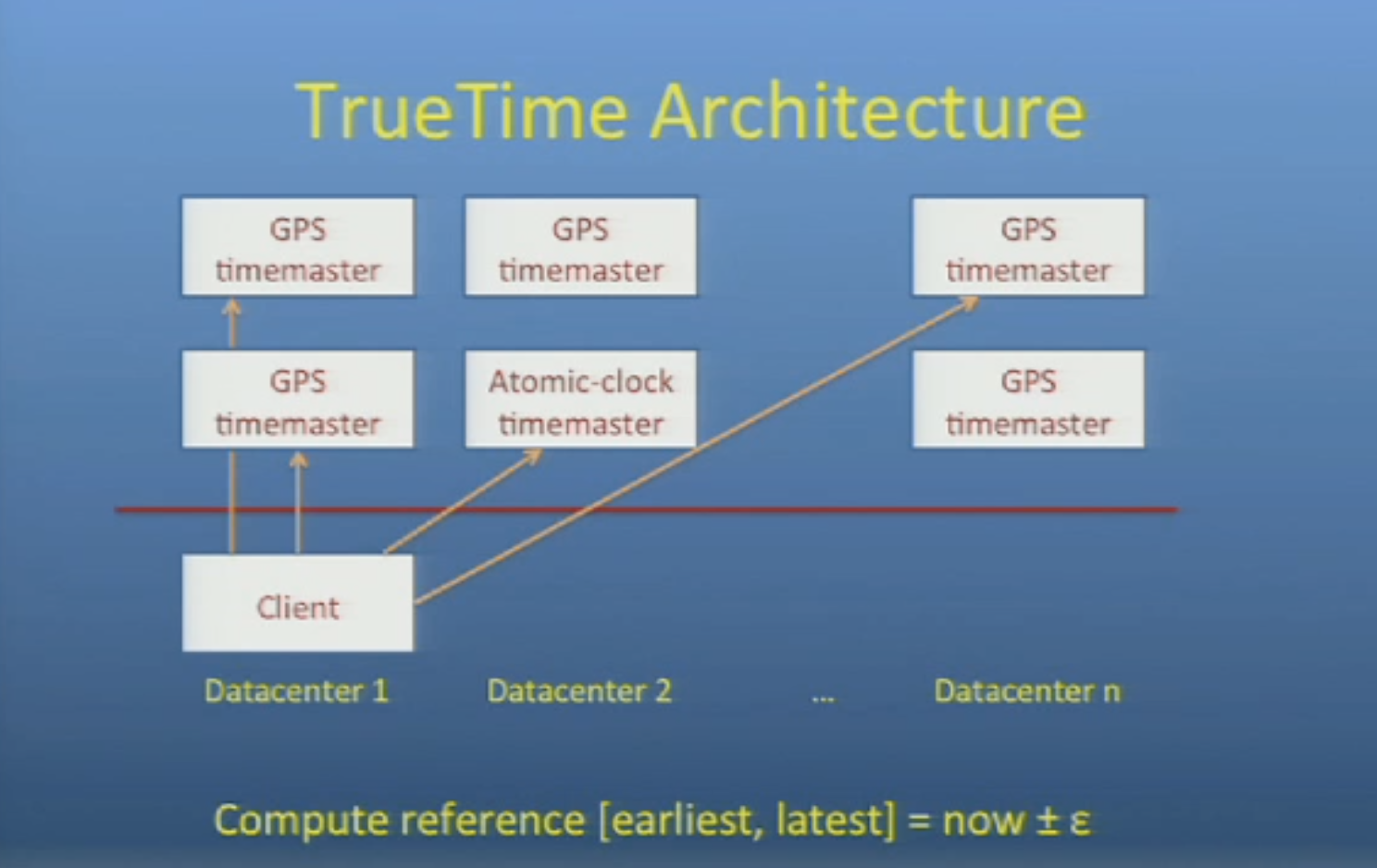True Time 暴露的接口如下

Method Returns
TT.now() TTinterval: [earliest, latest]
TT.after(t) true if t has definitely passed
TT.before(t) true if t has definitely not arrived

## 6 NTP

NTP(network time protocol) 最早由D. Mills在1981年提出, 之后成为计算机时间同步的重要协议.

NTP 分为NTP 和 SNTP, 前者基于osi更底层的协议icmp或者ggp(gateway-gateway protocol), 后者基于tcp, 实现思路类似但是具体方法类似(算round trip time), 也有很多个版本, 不同版本间的数据格式可能不太一样. 关于NTP的详细算法描述可以参考, RFC 5905 和 RFC 7822.

NTP server 一般依赖一个外部时钟源(reference clock), GPS and radio time and frequency broadcasts are commonly used as NTP reference clocks.

ntp server 类似于 dns server 都是有一定层级的, 结构大概如下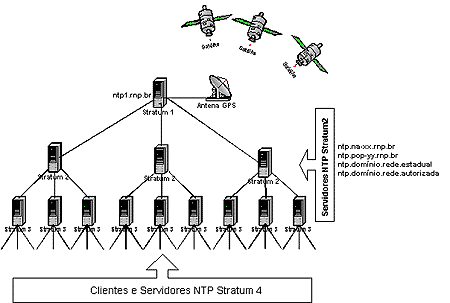ntp有几个跟时间同步的精度有关系关键的指标:

• Stratum: The Stratum of a NTP server denotes its level in the timing hierarchy. A stratum 1 NTP server obtains time from an external timing reference. Stratum 2 devices obtain time from stratum 1 devices and pass timing information to the next level and so on.

• Jitter: The jitter associated with a timing reference indicates the magnitude of variance, or dispersion, of the signal. Different timing references have different amounts of jitter. The more accurate a timing reference, the lower the jitter value. Jitter is usually measure in milliseconds.

• Offset: Offset generally refers to the difference in time between an external timing reference and time on a local machine. The greater the offset, the more inaccurate the timing source is. Synchronised NTP servers will generally have a low offset. Offset is generally measured in milliseconds.

• Delay: Delay in a NTP server describes the round-trip delay or latency of a timing message passed from client to server and back again. The delay is important so that network delays can be calculated and accounted for by a time client.

linux

$ntpdc -c loopinfo offset: -0.000060 s frequency: 2.822 ppm poll adjust: 30 watchdog timer: 1942 s  macos $ sntp -d time.asia.apple.com
sntp 4.2.8p10@1.3728-o Tue Mar 21 14:36:42 UTC 2017 (136~2590)
kod_init_kod_db(): Cannot open KoD db file /var/db/ntp-kod: No such file or directory
handle_lookup(time.asia.apple.com,0x2)
move_fd: estimated max descriptors: 4864, initial socket boundary: 20
sntp sendpkt: Sending packet to 17.253.116.125:123 ...
Packet sent.
sock_cb: time.asia.apple.com 17.253.116.125:123
2019-08-16 11:50:12.990601 (-0800) +0.000296 +/- 0.000380 time.asia.apple.com 17.253.116.125 s1 no-leap


David L. Mills的论文中还描述说到, 在21世纪初已经能做到纳秒级别 的时间同步精度. 时间同步的精度应该不是大问题.

## 7 Logical Clocks and Vector Clocks

Logical Clocks (Lamport Clocks, LC) and Vector Clocks (Vector Time, VC), 本质上都是 logical clocks, vector clock扩展了logical clocks, 通过使用额外的存储(drawback of vector clocks), 多增加了一个没有Logical clocks没 有的属性.

LC VC 算法概览如下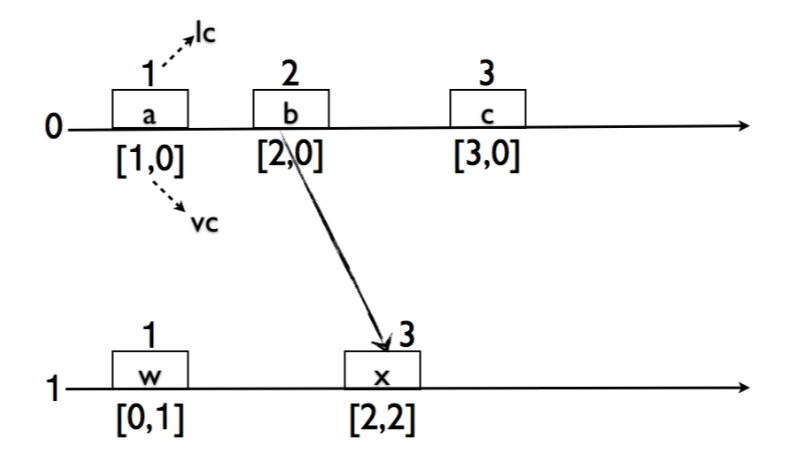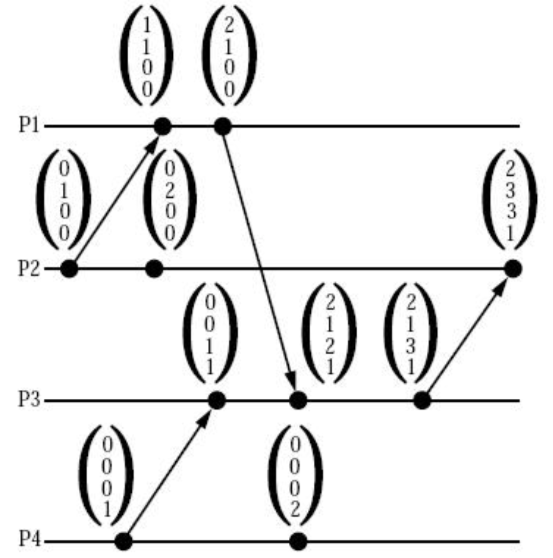vector clock的大小比较的定义,

For two time vectors

However, the following claims are not true:

LC/VC有比较明显的缺点:

• 如果集群中有两个节点长时间(永远)没有交互, 那么他们的逻辑时钟计数会相差非常大 (unbounded), 后续提出的HLC很重要的一点就是解决了这个问题.
• 不能体现出事件物理时间上的差距, 如果需要知道比如”3天前”的事件, 逻辑时钟就没法 做到这个

## 8 Hybrid Logical Clock

HLC 综合了物理时间, 基于NTP不需要额外的硬件, 同时加上了一部分逻辑时钟, 所以称之 为hybrid, HLC算法 克服了LC VC的一些缺点, 使得clock具有物理时间的特性, 同时也能解 决绝大部分分布式系统中的时钟问题, 可以作为一个比较好的True Time替代品.

HLC时钟逻辑部分一般是16位, 物理部分一般是43-48位, 物理部分时间单位为毫秒, 一般来 说这个格式也不需要留保留位(高位若干位), 因为一般都是要持久化的, 如果设置了保留位 整个时间戳的处理流程都要为处理保留为留好扩展空间.

HLC时钟选择64位, 据论文里说是为了保持NTP time format一致(方便直接取ntp的高位的若 干位?), 但是我个人认为实际实现 中并没有太大关系, 主要是为了让整个时间简单, 并且 其中物理部分和逻辑部分的位数是可以在具体实现时取合理的值(比如逻辑部分区20位, 物 理部分区44位).

63                                                        15              0
+---------------+-----------------------------------------+---------------+
| reserved(opt) |                 physical                |   logical     |
+---------------+-----------------------------------------+---------------+
l                            c


HLC算法表述如下: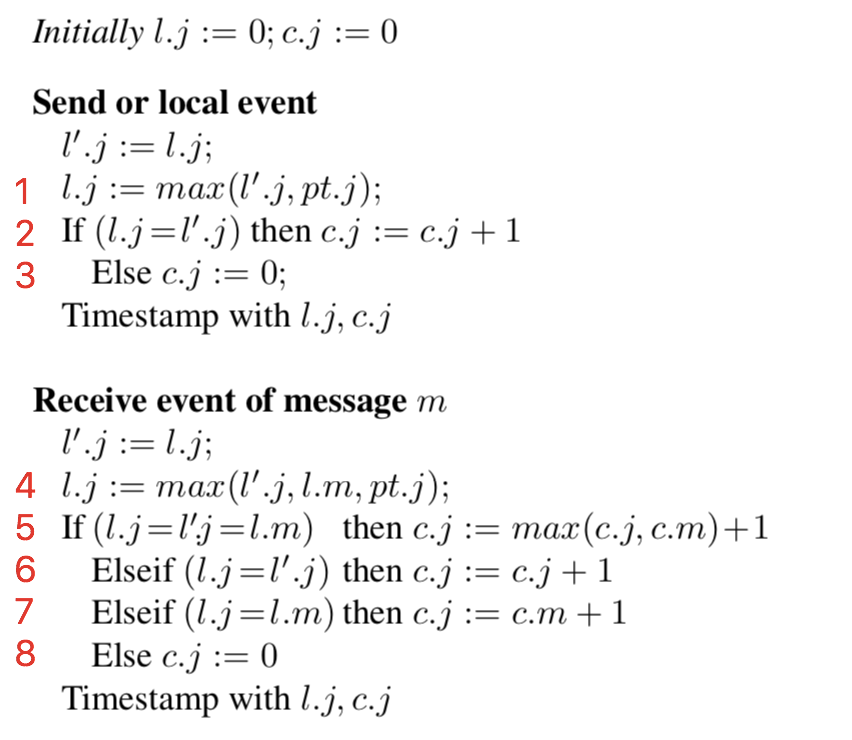• pt: 当前机器的时间, 由ntp进行同步
• l: hlc时钟的物理部分
• c: hlc时钟的逻辑部分
• m: 请求

1. 发起请求方的hlc时钟, 按照ptl最大值作为hlc的物理部分, 如果hlc物理部分不 变, 则自增c
2. 请求接收方, 接收到请求之后, 通过比物理时间较来检查是否要更新本地的hlc时钟
• 如果所有的物理时间都一样, 那么接收方的c就取发起方和接受方的最大c+1
• 如果请求接收方的hlc时钟里的物理时间最大, 则自增接收方的c
• 如果发起方的hlc时钟物理部分大(remote的时间比当前机器时间超前), 则更新本地HLC 时钟, 值为发起方的hlc时钟+1 (物理部分直接赋值, 逻辑部分+1).
• 如果接收方的pt是所有物理时间里最大, 说明本地机器时间已经向前, 并且本地的l >= 发起方的l, c = 0.

Theorem 1. For any two events $e$ and $f$,

Theorem 2. For any event $f$, $l.f ≥ pt.f$

Theorem 3. $l.f$ denotes the maximum clock value that $f$ is aware of. In other words,

Theorem 4. For any event $f$,

Corollary 1. For any event $f$, $|l.f−pt.f|≤ε$

Corollary 2. For any event $f$, $c.f≤|{g:g\text{ hb }f∧ l.g = l.f)}|$

Corollary 3. For any event $f$, $c.f≤N*(ε+1)$

Corollary 4. With assumption made below $c.f ≤ ε/d + 1$. Assumption to reduce the bound on $c$ further: We assume that the time for message transmission is long enough so that the physical clock of every node is incremented by at least $d$, where $d$ is a given parameter.

Theorem 1, 2 and 3 imply the monotonicity of HLC. Corollary 1, 3 and 4 show that $|l - pt|$ and $c$ are bounded.

### 8.1 HLC offset

note: 最差情况下

1. node1 给node2 发送了请求, 使用$l_1 = pt_1 = x$, $c_1 = 0$
2. node2 接收到请求, 根据HLC算法描述, $l_2 = l_1 = pt_1 = x$, $c_2 = 1$, $pt_2 = 0$ 这个时候$l_2 - pt = x$
3. node2 给node3 发请求, 使用 $l_2 = l_1 = pt_1 = x$, $c_2 = 2$, $pt_2 = 0$
4. node3 接收到请求, 使用 $l_3 = l_2 = l_1 = pt_1 = x$, $c_3 = 3$, $pt_3 = 0$
5. node2 给node3 发请求, 使用 $l_2 = l_1 = pt_1 = x$, $c_2 = 3$, $pt_2 = 0$
6. node3 接收到请求, 使用 $l_3 = l_2 = l_1 = pt_1 = x$, $c_3 = 4$, $pt_3 = 0$
7. 重复步骤3 4 5 6

### 8.2 Recovery

HLC 中有一条比较重要的性质, 这个按照HLC算法的描述其实是显而易见的.

pt <= l


|l - pt| <= ε


### 8.3 Implementation

HLC的实现比较简单, github有一个现成的实现

github.com/cockroachlabs/cockroachdb

cockroach/pkg/util/hlc/hlc.go


1. pt往回跳变需要观察
2. ptl的diff要控制在maxOffset内, 如果相差太大 系统可能工作就不正常了
3. c部分的溢出检查, 有两种方式:
1. 直接”进位”到 l上, 但是这么操作可能会违反HLC算法中l - pt <= e的属性, 需 要谨慎操作
2. 检测最大值, 如果到达最大值, 当做异常处理

第2种处理方式比较可取, 也有理论验证, 如果有异常了 应该就是ntp时间同步出问题了, 就应该当做异常情况处理.

TBD

TBD